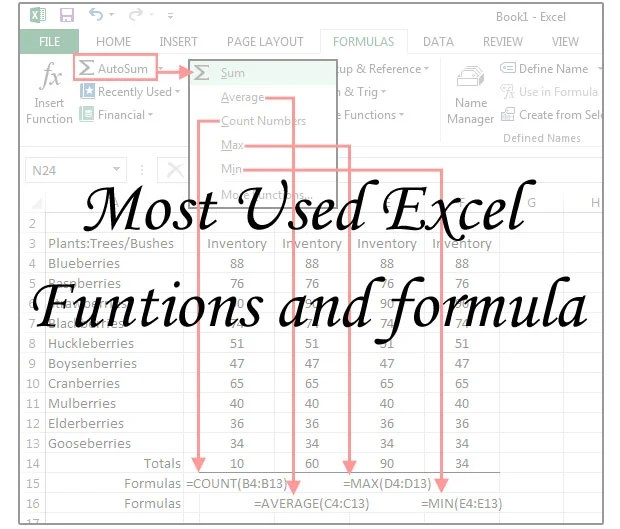Hello Guys today i will list out all the important function and formula in excel, which can be used in daily basis.

A formula is an expression which calculates the value of a cell. Function is in-built formula in excel.

### General Formula

MAX()To get the maximum value from a set of numbers, use the MAX function.=MAX(B5,C5)
MIN()To get the minimum value from a set of numbers, use the MIN function.=MIN(C6:G6)
COUNTIF()Countif is used to give condtion while counting the list of numbers.=COUNTIF(range, criteria)
COUNT()To count the number of cells that are not blank, use the COUNT function=COUNT(A1:A10)
COUNTA()The COUNTA function counts non-blank cells that contain numbers or text. It also counts space.=COUNTA(A1:A10)
LEN()If you need to count the total characters in a cell, you can do so using the LEN function=LEN(A1)
TRIM()The Excel TRIM function strips extra spaces from text.=TRIM(A1)
IF()IF(Something is True, then do something, otherwise do something else)=IF(A1>=60%,”First Class”,IF(A1>=45%,”Second Class”,”Third Class”))
SUMIF()It calculates the set of number with condition provided=SUMIF(range, criteria, sum_range)
SUMIFS()The SUMIFS function adds all of its arguments that meet multiple criteria.=SUMIFS(sum_range, criteria_range1, criteria1, [criteria_range2, criteria2], …)
AVERAGEIF()Calculates the average of the set of numbers related with respect to the provided condition.=AVERAGEIF(range, criteria, average_range)
LARGE()To return the largest values in a set of data with criteria, you can use the a formula based on the LARGE and IF functions.=LARGE(values,n)
EXACT()If you need to two text strings in Excel to determine if they’re equal, you can use the EXACT function. If the two strings are identical, EXACT will return TRUE. If not, EXACT will return FALSE=EXACT(text1, text2)
COUNTIFS()The COUNTIFS function applies criteria to cells across multiple ranges and counts the number of times all criteria are met.=COUNTIFS(range1,criteria1,range2,criteria2)
CONCATENATE()Concatenates two cell data into one cell.=CONCATENATE()
NOW()Prints current date and time.=NOW()
SMALL()Return this smallest value from the selected range.=SMALL(range,nth)
SUBSTITUTE()It replaces one set of characters with another in a specified cell or a text string=SUBSTITUTE(text, old_text, new_text, [instance_num])
TEXT()Converts a number or a date into a test string in the specified format.=TEXT(value, format_text)
VALUE()Converts a text string to a number.=VALUE(text)
UPPER()Converts lowercase to uppercase.=UPPER(“value”)
LOWER()Converts uppercase to lowercase.=LOWER(“value”)
PROPER()Capitalizes the first letter of each word and converts all other letters to lowercase.=PROPER(“name”)

### Mathematical Formula

 SUM() Calculate the sum of the selected range or number =SUM(A1, B1) AVERAGE() Calculate the average of the selected range or number =AVERAGE(B5:D5) SQRT() Calculates the Square root of the number. =SQRT(No) ROUND() It rounds of the number to the specified number of digits after decimal. =ROUND(3.15454424242,3) RAND() This formula generates random number inside that cell =RAND() RANDBETWEEN() The Excel RANDBETEEN function returns a random integer between given numbers =RANDBETWEEN (bottom, top)

### Logical Formula

 AND() Returns TRUE if all of the arguments evaluate to TRUE, FALSE otherwise. =AND(logical1, [logical2], …) OR() Returns TRUE if at least one of the arguments is TRUE. =OR(logical1, [logical2], …) NOT() Sum() =NOT()

### Financial Formula

 Simple Interest Calculate Simple interest. =principal*rate*term PPMT() To calculate the principal portion of a loan payment in a given period, you can use the PPMT function. =PPMT(rate,period,periods,-loan) NPER() To calculate the number of payment periods for a loan, given the loan amount, the interest rate, and a periodic payment amount, you can use the NPER function. =NPER(rate,payment,-loan) PMT() To calculate a loan payment amount, given an interest rate, the loan term, and the loan amount, you can use the PMT function. =PMT(rate,periods,-amount) RATE() To calculate the periodic interest rate for a loan, given the loan amount, the number of payment periods, and the payment amount, you can use the RATE function. =RATE(periods,payment,-amount)*12 FV() To calculate compound interest in Excel, you can use the FV function. =FV(rate,nper,pmt,pv)

### Miscellaneous

 GCD() To generate the ratio of two numbers to each other (e.g. 4:3, 16:9, etc.), you can do using division, the GCD function. =num1/GCD(num1,num2)&”:”&num2/GCD(num1,num2) IFERROR() To catch errors that a formula might trigger in a worksheet, you can use the IFERROR function to display a custom message. =IFERROR(formula,value_if_error) MODE() To find the most frequently occurring number in a range. =MODE(range) INDEX() If you need to reverse a list, use index funtion. =INDEX(list,COUNTA(list)+ROW(list)-ROW(),1) SEARCH() When SEARCH finds a match, it returns the position of the match in the cell being searched =SEARCH(“search text”,range) Cuberoot() Returns Cuberoot of a number =number^(1/3) Cuberoot() Returns Cuberoot of a number =number^(1/3) Cuberoot() Returns Cuberoot of a number =number^(1/3) Cuberoot() Returns Cuberoot of a number =number^(1/3) Cuberoot() Returns Cuberoot of a number =number^(1/3) Cuberoot() Returns Cuberoot of a number =number^(1/3) Cuberoot() Returns Cuberoot of a number =number^(1/3)

I hope I have covered most used function and formula’s, if you more you would like to add just add to comment. I will add more in the future.

This site uses Akismet to reduce spam. Learn how your comment data is processed.